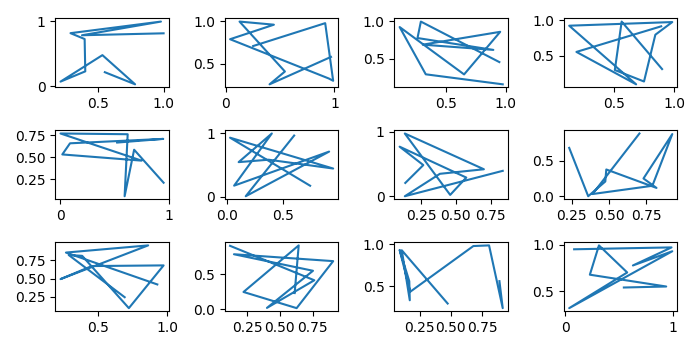# How should I pass a matplotlib object through a function; as Axis, Axes or Figure?

To pass a matplotlib object through a function; as Axis, Axes or figure, we can take the following steps −

• Set the figure size and adjust the padding between and around the subplots.
• In plot() method, plot x and y data points at axes ax.
• In profile() method, create a figure and a set of subplots. Iterate the axes and pass in plot() method to plot the figure.
• Call the profile() method with 3 rows and 4 columns.
• To display the figure, use show() method.

## Example

import numpy as np
from matplotlib import pyplot as plt

plt.rcParams["figure.figsize"] = [7.00, 3.50]
plt.rcParams["figure.autolayout"] = True

def plot(ax, x, y):
ax.plot(x, y)

def profile(rows, cols):
fig, axes = plt.subplots(rows, cols)
for ax in axes:
for a in ax:
plot(a, np.random.rand(10), np.random.rand(10))

profile(3, 4)
plt.show()

## Output

It will produce the following output